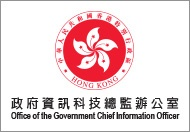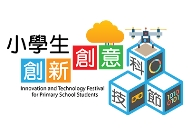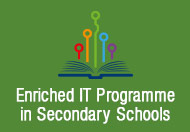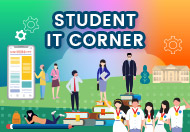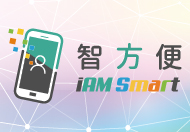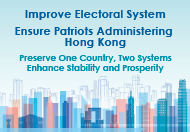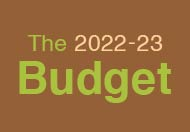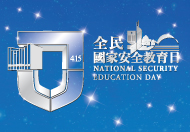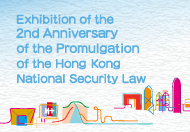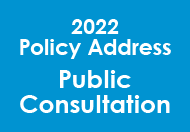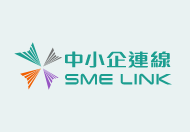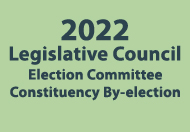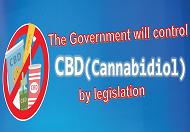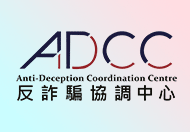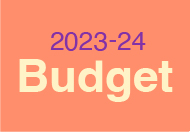## School Coding Festival

Objective:
To encourage students to learn programming and exercise computational thinking to solve mathematical problems efficiently.

Description:
The school will organise an intra-school coding festival, including a student competition to solve a set of mathematics problems. Mathematics and computer science often go hand in hand. A great deal of applied mathematics is performed on computers such as solving large systems of equations and approximating solutions to differential equations for which no closed formula exists. The competition will require the students to solve different mathematical problems, such as prime factorisation, calculation of binomial coefficients, generating permutations, solving geometric problems (e.g. determine whether a point is within a circle or triangle, calculating the overlapping areas of two polygons), etc. Different algorithms can solve the mathematical problems but the one that executes in the shortest time with the same configuration of computers will win. The school will showcase their winning algorithms in a coding festival open to other schools and the public to visit.

 Hardware PC / laptop / tablet computer Software app simulator Common programming languages like Javascript / C# / Python / Java / C++ / Swift Major Activities Theoretical session covering: Common daily life Mathematics problems such as optimisation, classification, etc., and how computational thinking helps Algorithm design such as "divide and conquer", "greedy method", "dynamic programming", "linear programming", etc. Practical sessions covering: Coding, testing, debugging, tuning Develop applications with specific requirements for examples : Develop an app to count the number of occurrences of a given substring in a paragraph Develop an app to compute Pi with the given formula, make a textbox for a user to input the number of iterations for the computation Develop a drawing app to let a user draw on the screen. The colour of each line will depend on the line sequence Develop an app for drawing a triangle by dragging three dynamic points in a canvas and display the value of the three angles in degree The school will arrange an intra-school competition for solving different mathematical problems for all classes so as to raise the learning atmosphere of IT in the school. The one that executes in the shortest time with the same configuration of computers will win. The school will showcase their winning algorithms in a coding festival open to other schools and the public to visit. Learning Objective(s) Enhance students' computational thinking to solve these mathematical problems Raise students' interest in coding and mathematics Encourage students to express daily life problems in mathematical perspective Duration Theoretical session : 4 hours Practical session : 8 hours Competition : 1 week Coding festival : 1 day Difficulty Low Target Level S1 - S6 Target No. of Students About 20 students per workshop4 students in a groupIntra-school competition to open to all students

Site Map